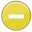#miscalculate 中文解釋 wordnet sense Collocation Usage Collins Definition
Verb
/misˈkalkyəˌlāt/,Font size:miscalculating, present participle; miscalculated, past tense; miscalculated, past participle; miscalculates, 3rd person singular present;
1. Calculate (an amount, distance, or measurement) wrongly

2. Assess (a situation) wrongly
• - the government has seriously miscalculated the effect of privatization
• - you miscalculated if you imagined I'd fallen for your little scheme

1. judge incorrectly; "I had misestimated his determination"
2. calculate incorrectly; "I miscalculated the number of guests at the wedding"
3. (miscalculation) a mistake in calculating
4. To incorrectly calculate; to make a gross error in judgement
5. (Miscalculation) Hastiness may cause decreased accuracy when entering Aim Mode.